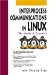# D.2. Sample Program for Profiling

A selection sort program is used to demonstrate the profiling process. A selection sort is a general-purpose sort that is a variation of an exchange sort. Basically, this sort passes through a list of elements and finds the index (location) of the smallest (least) element. It exchanges the data at this index with the data stored in the first element of the list. It then repeats the selection process with the remaining list to find the second smallest element, and exchanges the data at this index with the data stored in the second element of the list. The process is repeated until the list is ordered.

There are numerous ways to code a selection sort. Below is a C++ version of this sort.

```File : ss.cxx
#include
using namespace std; // Function prototypes
void Get_Data ( int [], int );
void Display ( int [], int );
+ void Do_S_Sort ( int [], int );
int Find_Least( const int [], int, int );
int Compare ( const int & , const int & );
void Swap ( int &, int & );
int
10 main( ) {
const int max = 10;
int List[max];
Get_Data ( List, max ); // Obtain data
cout << "Initial list" << endl;
+ Display ( List, max ); // Show it
Do_S_Sort( List, max ); // Sort it
cout << "Sorted list" << endl;
Display ( List, max ); // Show it again
return 0;
20 }
// Obtain data to sort from standard input
void
Get_Data(int a[], int n) {
cout << "Please enter " << n << " integers" << endl;
+ for(int i=0; i < n; ++i)
cin >> a[i];
}
// Display the current contents of list
void
30 Display(int a[], int n) {
for(int i=0; i < n; ++i)
cout << " " << a[i];
cout << endl;
}
+ // Do the Selection Sort, Display after each pass
void
Do_S_Sort( int a[], int n ){
int index;
for (int i=0; i < n-1; ++i){
40 index=Find_Least( a, i, n );
if ( i != index )
Swap( a[i], a[index] );
cout << "After pass " << i+1 << " : ";
Display( a, n );
+ }
}
// Find the index of the least element in list
int
Find_Least( const int a[], int start, int stop ){
50 int Index_of_Least = start;
for (int i=start+1; i < stop; ++i )
if ( Compare(a[i], a[Index_of_Least]) )
Index_of_Least = i;
return Index_of_Least;
+ }
// Compare two data elements
int
Compare( const int &a, const int &b ){
return ( a < b );
60 }
// Exchange two data elements
void
Swap( int &a, int &b ){
int temp;
+ temp = a;
a = b;
b = temp;
}
```

As shown, six functions are used to implement the sort

FUNCTION

PURPOSE

Get_Data

Obtains the data to be sorted from standard input.

Display

Prints the current contents of the list to standard output.

Do_S_Sort

Performs the selection sort routine.

Find_Least

Traverses a portion of the list to find (and ultimately return) the index of the smallest element.

Compare

Compares two list elements and returns a nonzero value if the first element is less than the second element.

Swap

Exchanges the data for two elements in the list.

At first glance, using so many functions for such a simple algorithm may seem to be overkill. However, coding some statements (such as the compare in line 52) as a function call will facilitate referencing when generating profile information with gprof .

A good portion of the work done by the selection sort is actually performed by the Find_Least function (see line 49). This function is passed the list and two integer values. The first integer value is the starting point for the search through the remainder of the list. The second value is the size of the list (which in this case reflects its end or stopping point). This function works by storing, in the variable Index_of_Least , the index of the element having the smallest value. Initially, this is the index of the current start of the list (see line 50). It then uses a for loop to pass through the reminder of the list, checking each location against the current smallest value to determine if it has found a lesser value. Each check is carried out by the Compare function. If the value is less, the index (not the value) of the location is stored. When the loop finishes, the index of the element with the smallest value is returned to the calling function. If the returned index is not the index of the current head of list, the two values are exchanged by calling the Swap function. If the current value is already in order, no exchange is needed.Interprocess Communications in Linux: The Nooks and Crannies
ISBN: 0130460427
EAN: 2147483647
Year: 2001
Pages: 136